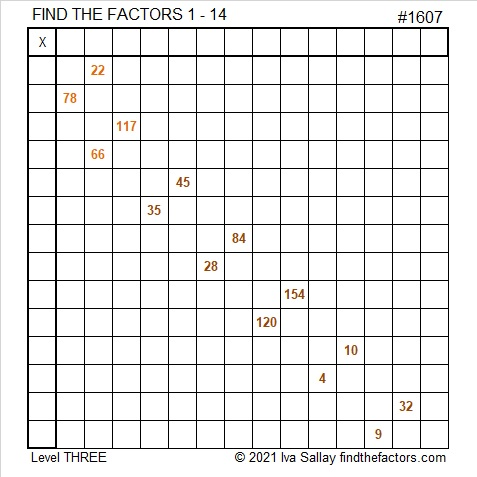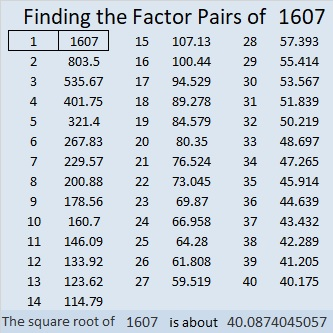# 1607 Shillelagh

Contents

### Today’s Puzzle:

A Shillelagh is an Irish wooden walking stick. This Shillelagh is keeping with our Saint Patrick’s Day theme, but it is a Find the Factors 1 to 14 puzzle.  Brutal! It will be a whole lot less tricky for you to solve because I made it a level 3 puzzle: The logic needed to solve the puzzle is built in. Just start with the clue at the top of the puzzle and work your way down cell by cell until you have found all the factors. So crack on!Print the puzzles or type the solution in this excel file: 14 Factors 1604-1612.

### Factors of 1607:

• 1607 is a prime number.
• Prime factorization: 1607 is prime.
• 1607 has no exponents greater than 1 in its prime factorization, so √1607 cannot be simplified.
• The exponent in the prime factorization is 1. Adding one to that exponent we get (1 + 1) = 2. Therefore 1607 has exactly 2 factors.
• The factors of 1607 are outlined with their factor pair partners in the graphic below.How do we know that 1607 is a prime number? If 1607 were not a prime number, then it would be divisible by at least one prime number less than or equal to √1607. Since 1607 cannot be divided evenly by 2, 3, 5, 7, 11, 13, 17, 19, 23, 29, 31, or 37, we know that 1607 is a prime number.

### More about the Number 1607:

1607 is the sum of two consecutive numbers:
803 + 804 = 1607.

1607 is also the difference of two consecutive numbers:
804² – 803² = 1607.

Did you notice what happened there? Try this next one:

1607² = 2582449.

1607²/2 = 1291224.5.

(1607-1291224-1291225) is a primitive Pythagorean triple.

Cool, isn’t it?

This site uses Akismet to reduce spam. Learn how your comment data is processed.